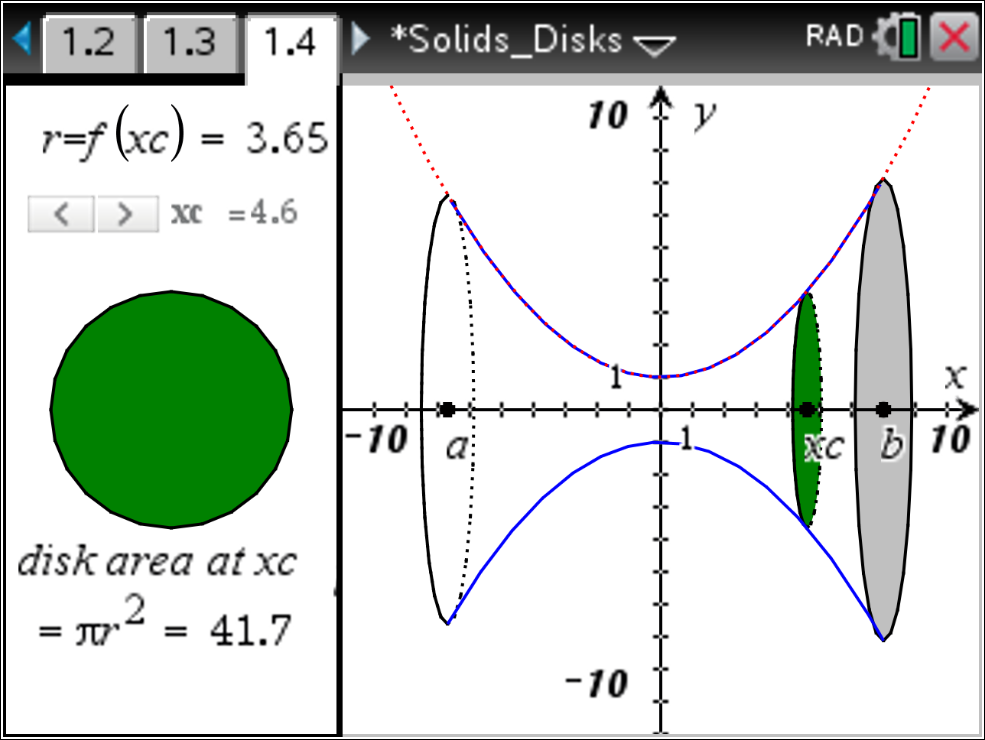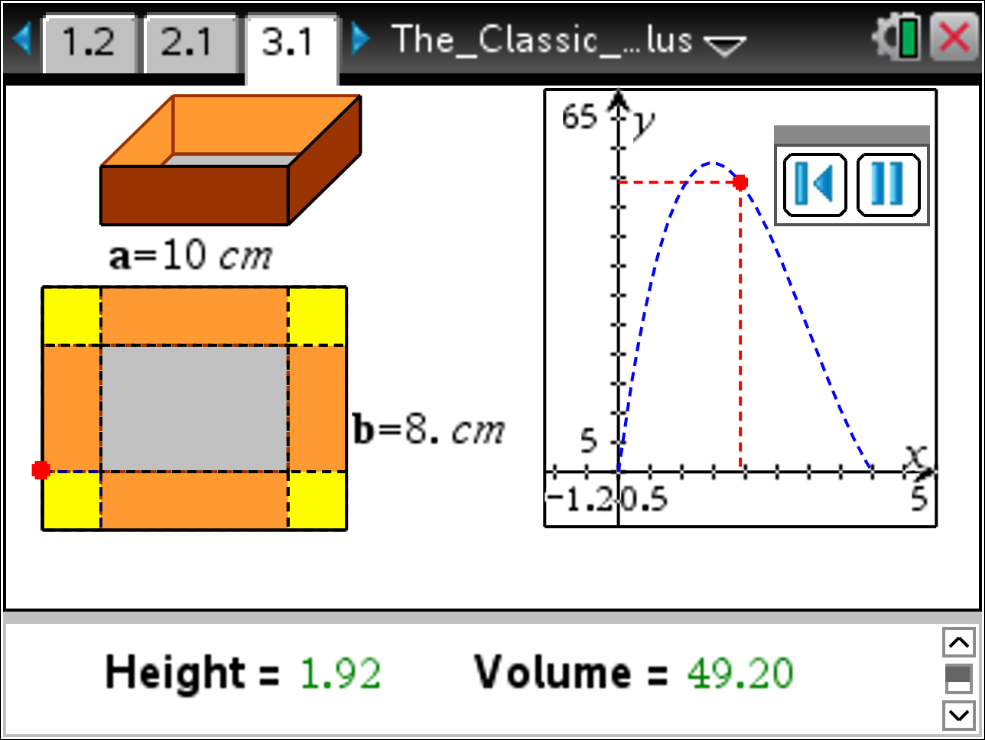## Five Free Activities For Teaching Calculus

Posted 07/05/2018 by Curtis Brown

Calculus is the study of rates of change and of lengths, areas and volumes. Often, students have a hard time grasping the concepts of calculus, and even more, making connections among them. Dynamic visualization is a good tool for helping students to visualize and interact with the mathematics. Being able to manipulate and see changes in relations can help to solidify concepts and relationships among them. These 5 lessons are some of our favorites for helping students to make connections in calculus.

1. Riemann Sums

In this activity, your students can quickly learn that for a continuous nonnegative function f, there is one interpretation of the definite integral f(x)dx from a to b, the area of the region R, bounded above by the graph of y = f(x), below by the x-axis, and by the lines x = a and x = b. This is perhaps one of the quickest ways to visualize Riemann sums and students can look at the left hand, right hand, and midpoint Riemann sums easily for many different functions and intervals.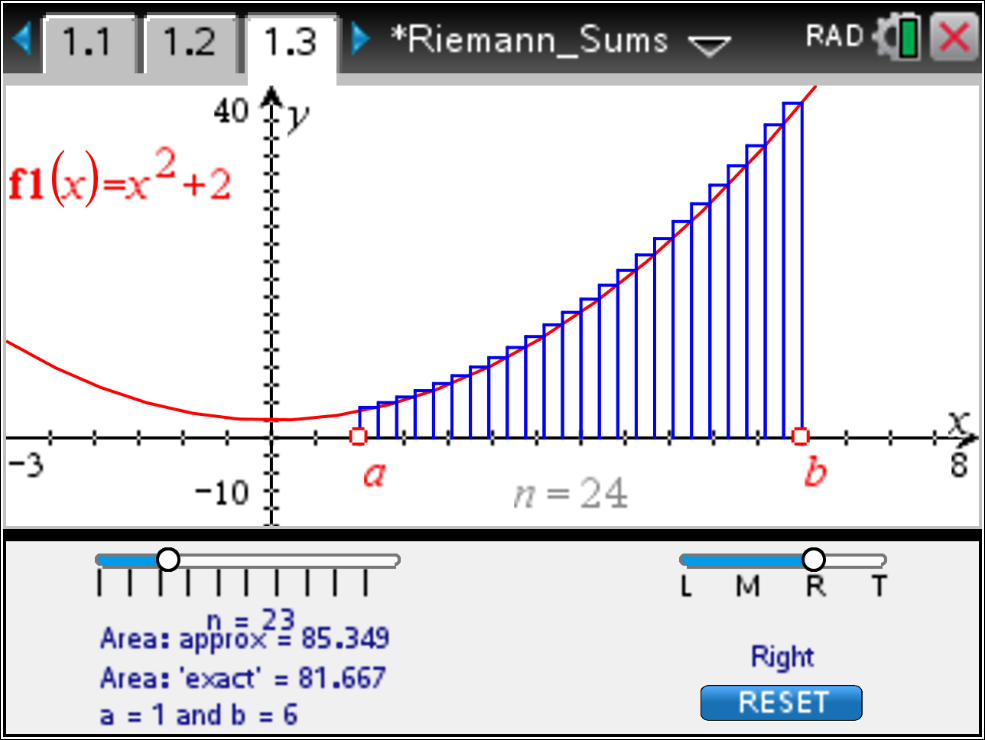You can view and download the full TI-Nspire activity on our website.

2. MVT for Derivatives

This lesson uses a graphical representation of the Mean Value Theorem (MVT) to demonstrate how the theorem relates information about the average rate of change of a function to an instantaneous rate of change.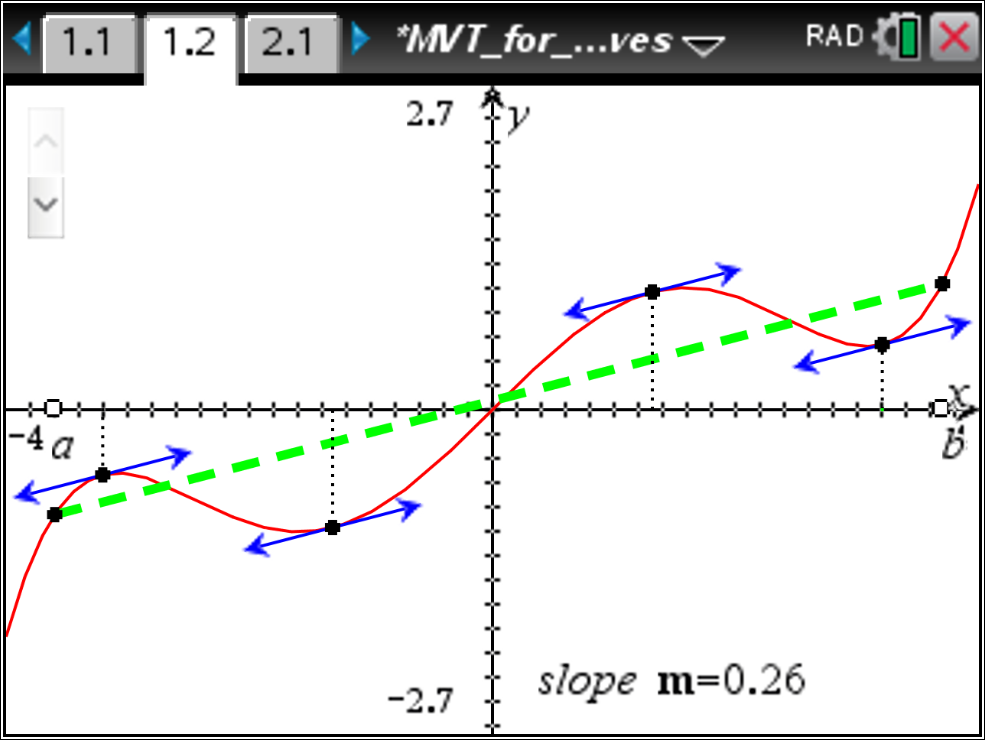You can view and download the full TI-Nspire activity on our website.

3. Filling the Urn

The Filling_the_Urn.tns TI-Nspire document provides for a graphical investigation of related rates. The physical context is the filling of an urn with liquid, depicted in a window on the left side of the illustration. The associated graph represents the height of liquid in the urn as a function of the amount of liquid in the urn. The shape of the urn determines the characteristics of this graph.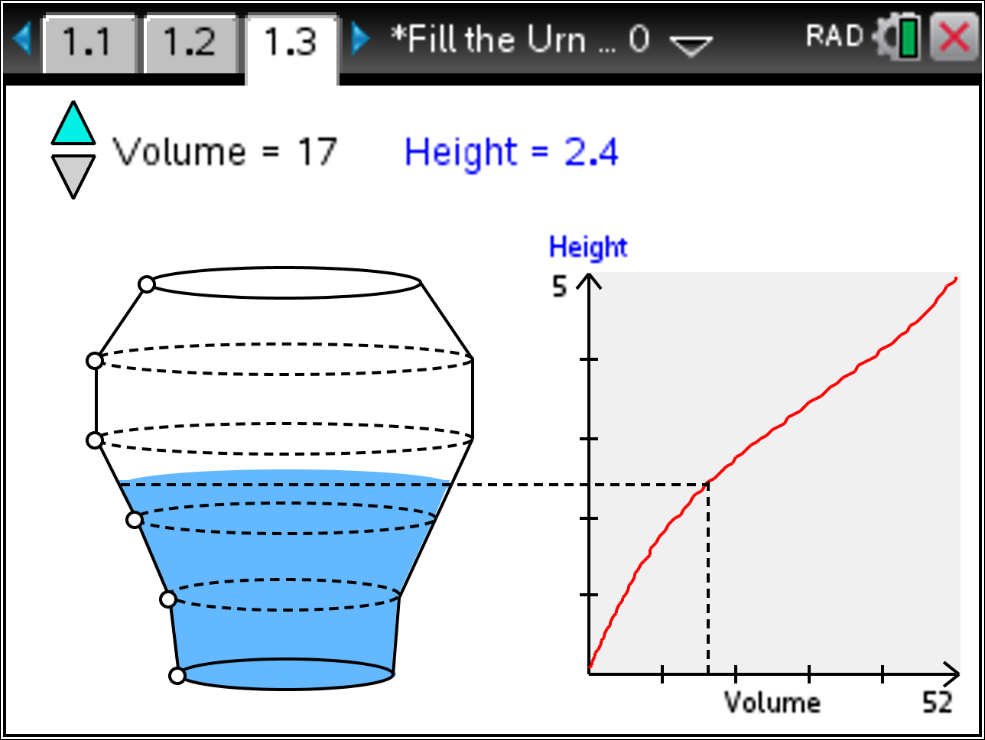You can view and download the full TI-Nspire activity on our website.

4. Visualizing Solids of Revolution – Disk Method

Students often have a difficult time visualizing the solids of revolution and how the volume and the integral are related. This interactive demonstration can help students make connections between the solid generated by revolving the function graph about the x-axis and disks centered on the x-axis, the radius of which correspond to the value of the function at that point.